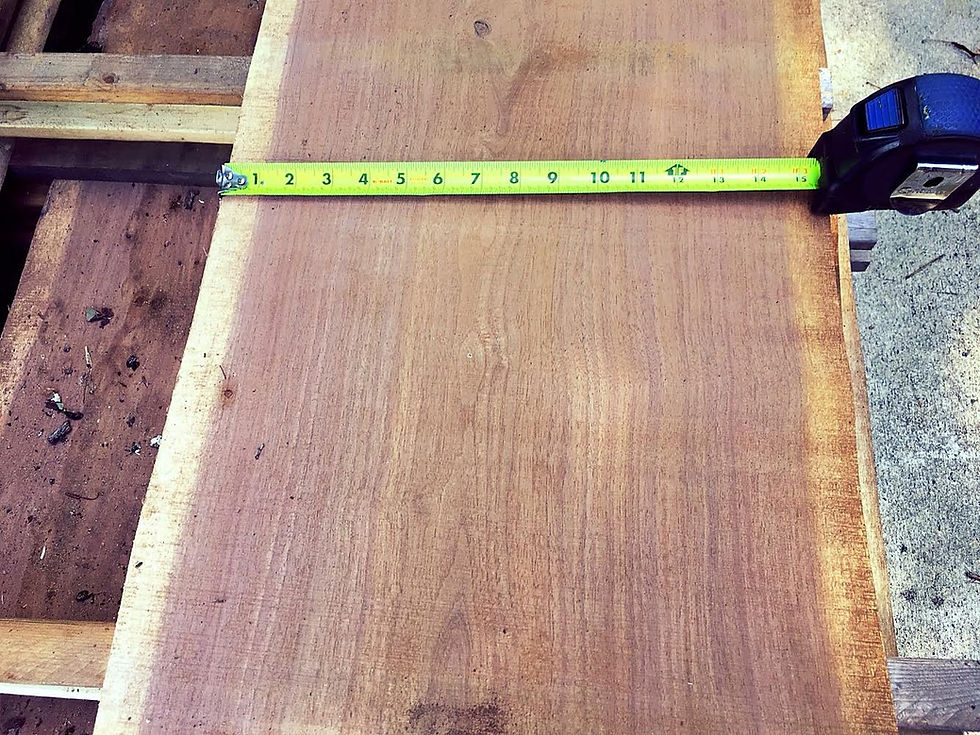top of page

# What Is A Board Foot?

Board Foot Measurement - A board foot (BF or bdft) is the basic unit of measurement for hardwood lumber. A board foot (or bdft) is 1 foot long x 1 foot wide x 1 inch thick. Its one square foot of boards, one inch thick, or one half square foot of boards, two inches thick. The formula for determining board feet is: height in inches multiplied by the width in inches multiplied by the length in feet divided by 12. (Height * Width* Length) /12. It's easy to remember. You see most of the formula when you look at the sign in the lumber yard. It says 2" x 4" x 8' Just multiply all the numbers together and remember to divide by twelve and you have board feet for any board. Board footage is a nominal, volume measurement and meant for rough lumber. If a board is less than 1 inch thick, then it is still calculated with a thickness of 1 inch.For example:

A standard 2x4-8 foot long is: 2 inch thick x 4 inch wide x 8 ft long /12 = 5.3 bdft

A board 12 inches wide 8 feet long and 1 inch thick is: 12 inch x 8 ft x 1 inch /12 = 8 bdft

A board 6 inches wide, 10 feet long and 1 inch thick is: 6 inch x 10 ft x 1 inch / 12 = 5 bdft

Credit for this article goes to Robert Milton, owner of Hobby Hardwoods Alabama.

Nathan,